×

Search anything:

# Grid Search

#### Machine Learning (ML)Get this book -> Problems on Array: For Interviews and Competitive Programming

Reading time: 30 minutes | Coding time: 10 minutes

Grid Search is a machine learning algorithm which is used to tune the hyperparameters of various machine learning algorithms like decision tree, SVM etc.

Its main aim to provide optimal value for hyperparametres to achieve better results. So it is generally used to perform hyperparameters optimization. As using grid search we will have best values for hyperparametres which led to best accuracy which can be achieved from that model. Since the accuracy or the performance of model is highly related to parametres values specified.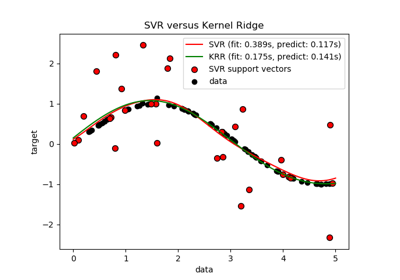# How it works?

The function of grid search is to provide best hyperparametres values for specfied algorithm with specified parametres. It performs comprehensive search over specified parametres for an estimator. But we have to also provide a evaluation metric system to grid search. On that basis of performance metric, grid search will generate best values. The grid search must be controlled by performance metric usually measured by cross validation on training set or evaluation on validation set.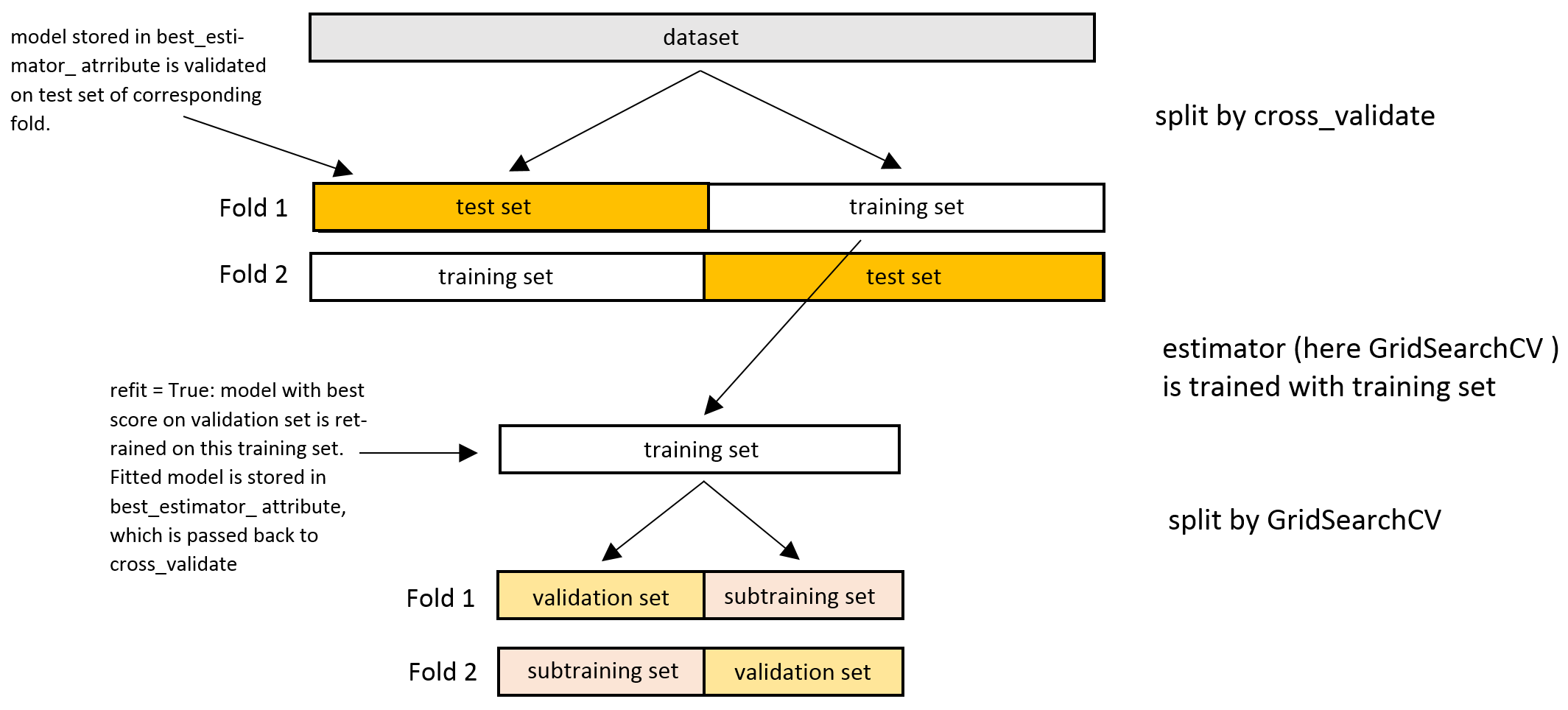We also have to specify a range of values with both upper and lower limit for specific parametres to grid search. This is mostly done in case when hyparametres values are continious. For example Random Forest classifier has 2 core continious hyperparametres which need tuning for good performance: max_depth and n_estimators.
So we will deicide a range of values for both of them like for max_depth we will use (3,4,5,6,8,9,10) and for n_estimators we use (100,150,200,250,400,500...). Grid search then train random forest model with set of pair and evaluate their performance and will give us the best pair.

Parametres in grid search:

• estimator: In this parametre we provide the defined machine learning model with self default hyperparameteres

• param_grid: In this we provide the hyperparameter we want to tune with set of range of values. It is provided in form of dictionairy with hyperparametre as key and range as values. This enables searching over any sequence of parameter settings.

• n_jobs: It refers amount of job to run in parallel. None means 1 and -1 means using all processors.

• cv: It determines the splitting strategy will be used for cross validation. None to use default 3-fold cross validation and an integer value to specify number of folds

• return_train_score: It used for computing training score and to get insights on how parametre settings would impact overfitting or underfittingtrade-off. False value mean will not include training score.

• scoring: It is used for evaluation of prediction on test set.

So basically we will define a machine learning model. Now to tune its hyperparametres for better performance we will use grid search. We will feed hyperparametres with certain range of values and our machine learning model to grid search. Grid Search will train each set of values we supplied and provides us the best values for our hyperparametres.

# Implementation

Now we will implement the Grid Search on a dataset for better understanding.
We will compare performance with and without using Grid Search

Now we will use a dataset which contains information about heart condition of patient and we have to predcit whether customer will get a heart attack or not.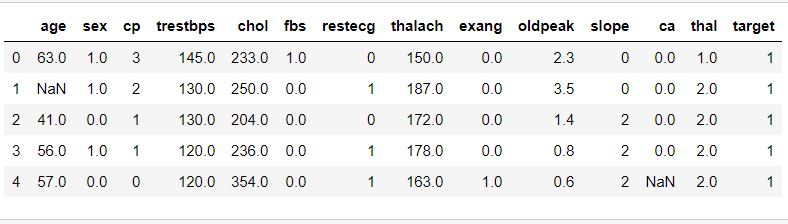Further we performed the necessary data processing and cleaning. Now we have to implement a machine learning model for response variable perdiction. For the purpose we chose Random Forest Classifier.

``````from sklearn.ensemble import RandomForestClassifier
clf=RandomForestClassifier()
``````

We will test the performance without using Grid Search first

``````clf.fit(x_train,y_train)
y_pred=clf.predict(x_test)

from sklearn.metrics import classification_report
print(classification_report(y_pred,y_test))
``````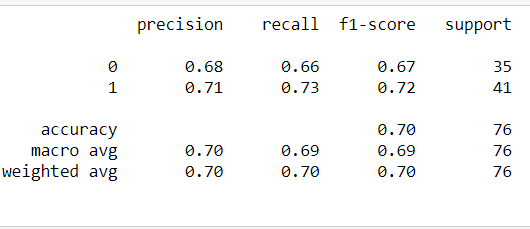Now we will implement Grid Search to tune the hyperparametres for better performance and compare the results.

``````from sklearn.model_selection import GridSearchCV

params={'max_depth':[3,4,5,6,8,9,10],
'n_estimators':[100,150,200,250,400,500,700,800,900,1000,1050],
'criterion':['gini','entropy']
}
grid_model=GridSearchCV(estimator=clf,param_grid=params,n_jobs=-1,cv=5,return_train_score=True,scoring='roc_auc')
grid_model.fit(x_train,y_train)
``````

For the purpose we used 3 core hyperparameters : max_depth, n_estimator and criterion. Now grid search will train model with each set of values, calculates the training score and will give us set of values providing best performance.

``````pd.DataFrame(grid_model.cv_results_)
``````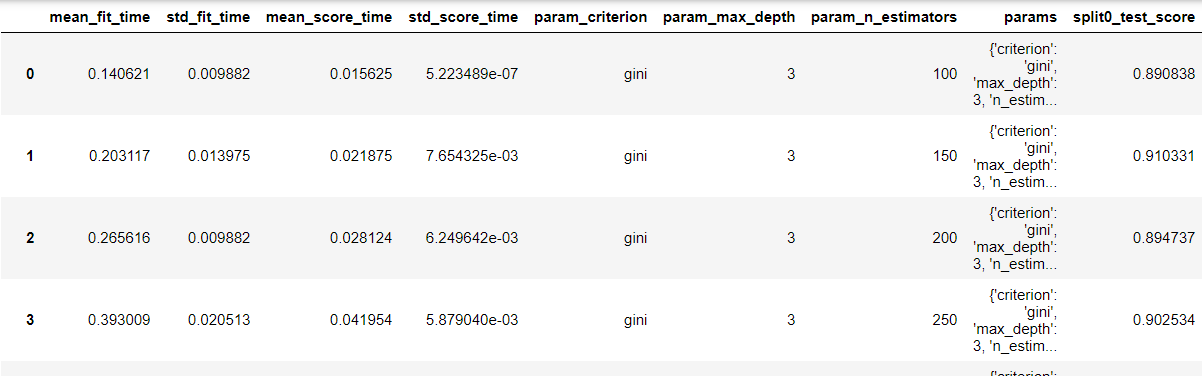``````grid_model.best_score_
>>>0.9004616005543435

grid_model.best_estimator_
``````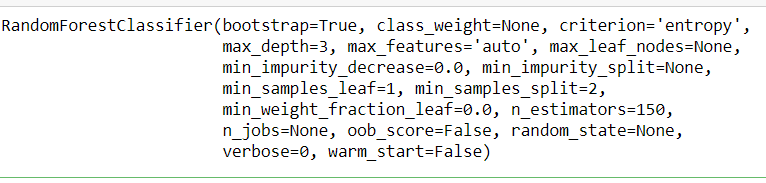``````clf_1=RandomForestClassifier(bootstrap=True, class_weight=None, criterion='entropy',
max_depth=3, max_features='auto', max_leaf_nodes=None,
min_impurity_decrease=0.0, min_impurity_split=None,
min_samples_leaf=1, min_samples_split=2,
min_weight_fraction_leaf=0.0, n_estimators=250,
n_jobs=None, oob_score=False, random_state=None,
verbose=0, warm_start=False)
clf_1.fit(x_train,y_train)
y_pred1=clf_1.predict(x_test)
print(classification_report(y_pred1,y_test))
``````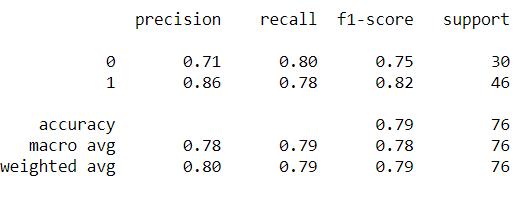There is decent different between accuracies. Accuracy achieved by using grid search is better than of earlier. This clearly shows how tuning the hyperparametre improves the performance of model.

# Limitations

• Time taken by grid search cv in larger dataset is very high
• When the dimensionality is high, more combination to search and hence more time taken
• Grid Search is not efficient in every case
• Grid Search considered as brute force method#### Harsh Bansal

Machine Learning Developer, Intern at OpenGenus | Bachelor of Technology Student in IT at Northern India Engineering College